# 2D/3D Linear Transformation

## 1 齐次坐标(Homogeneous coordinates)

In mathematics, homogeneous coordinates or projective coordinates, introduced by August Ferdinand Möbius in his 1827 work Der barycentrische Calcül, are a system of coordinates used in projective geometry, as Cartesian coordinates are used in Euclidean geometry.Figure 1: 两条铁轨在地平线处相交（图片摘自：http://www.songho.ca/math/homogeneous/homogeneous.html

$\begin{cases} X = x/w \\ Y = y/w \\ Z = z/w \\ \end{cases}$

## 2 二维线性变换

$\left[ \begin{array}{cc} a_{11} & a_{12} \\ a_{21} & a_{22} \end{array} \right] \left[ \begin{array}{c} x \\ y \end{array} \right] = \left[ \begin{array}{c} a_{11}x + a_{12} y \\ a_{21}x + a_{22}y \end{array} \right]$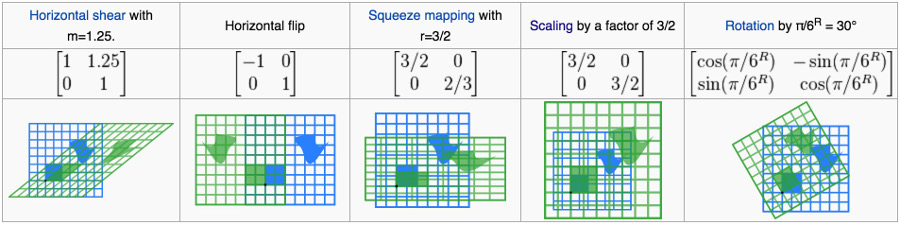Figure 2: 二维线性变换实例（蓝色为变换前图形，绿色为变换后图形，中心坐标用黑点表示）

### 2.1 二维线性变换组合

$v_2 = \mathbf{S} v_1, \; v_3 = \mathbf{R} v_2$

$v_3 = \mathbf{R}(\mathbf{S}v_1) = (\mathbf{RS})v_1$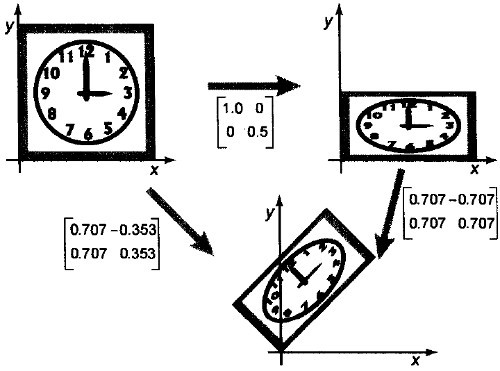Figure 3: 按顺序使用两个变换矩阵（先在垂直方向上压缩1/2，然后旋转45度），效果与一次使用两矩阵之积一样

### 2.2 二维线性变换分解（“旋转、缩放、再旋转”的乘积）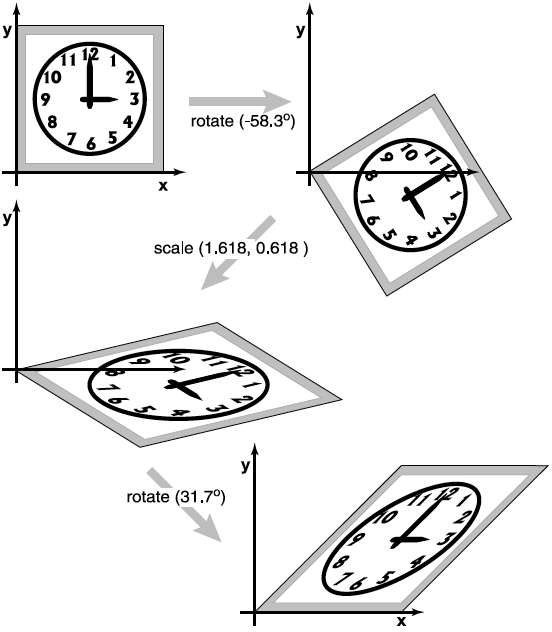Figure 4: 切变矩阵的奇异值分解（分解为旋转、缩放、再旋转）

\begin{aligned} \left( \begin{array}{cc} 1 & 1 \\ 0 & 1 \\ \end{array} \right) & = \left( \begin{array}{cc} 0.8507 & -0.5257 \\ 0.5257 & 0.8507 \\ \end{array} \right) \left( \begin{array}{cc} 1.618 & 0 \\ 0 & 0.618 \\ \end{array} \right) \left( \begin{array}{cc} 0.5257 & 0.8507 \\ -0.8507 & 0.5257 \\ \end{array} \right) \\ & = rotate(31.7^{\circ}) scale(1.618, 0.618) rotate(-58.3^{\circ}) \end{aligned}

### 2.3 二维线性变换（齐次坐标形式）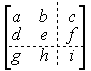Figure 5: 二维变换的变换矩阵（采用齐次坐标）

#### 2.3.1 平移变换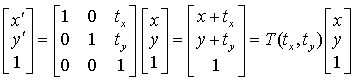Figure 6: 平移变换

#### 2.3.2 缩放变换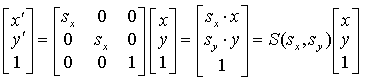Figure 7: 缩放变换

#### 2.3.3 旋转变换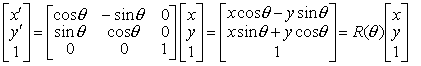Figure 8: 旋转变换

## 3 右手坐标系(Right-handed coordinate system)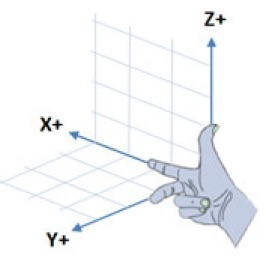Figure 9: 右手坐标系中三个坐标轴的关系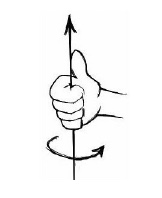Figure 10: 右手坐标系中，绕坐标轴旋转的“正方向”的规定

## 4 三维线性变换

$scale(s_x, s_y, s_z) = \left( \begin{array}{ccc} s_x & 0 & 0 \\ 0 & s_y & 0 \\ 0 & 0 & s_z \end{array} \right)$

### 4.1 平移、缩放等变换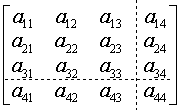Figure 11: 三维变换的变换矩阵（采用齐次坐标）

#### 4.1.1 三维平移变换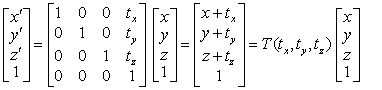Figure 12: 三维平移变换

#### 4.1.2 三维缩放变换

(1) 将平移到坐标原点处；
(2) 进行缩放变换；
(3) 将参考点 $$(x_f, y_f, z_f)$$ 移回原来位置。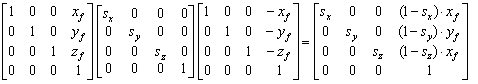Figure 13: 三维缩放变换矩阵

### 4.2 绕坐标轴的旋转

#### 4.2.1 绕z轴旋转Figure 14: 绕z轴旋转

$\begin{cases} x' = x \cos \theta - y \sin \theta \\ y' = x \sin \theta + y \cos \theta \\ z' = z \end{cases}$

$\left( \begin{array}{c} x' \\ y' \\ z' \\ 1 \end{array} \right) = \left( \begin{array}{cccc} \cos \theta & -\sin \theta & 0 & 0 \\ \sin \theta & \cos \theta & 0 & 0 \\ 0 & 0 & 1 & 0 \\ 0 & 0 & 1 & 1 \\ \end{array} \right) \left( \begin{array}{c} x \\ y \\ z \\ 1 \end{array} \right)$

#### 4.2.2 绕x轴旋转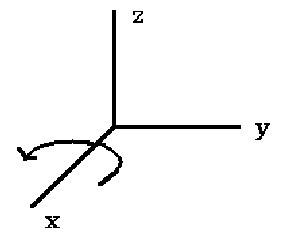Figure 15: 绕x轴旋转

$\begin{cases} y' = y \cos \theta - z \sin \theta \\ z' = y \sin \theta + z \cos \theta \\ x' = x \end{cases}$

$\left( \begin{array}{c} x' \\ y' \\ z' \\ 1 \end{array} \right) = \left( \begin{array}{cccc} 1 & 0 & 0 & 0 \\ 0 & \cos \theta & -\sin \theta & 0 \\ 0 & \sin \theta & \cos \theta & 0 \\ 0 & 0 & 0 & 1 \\ \end{array} \right) \left( \begin{array}{c} x \\ y \\ z \\ 1 \end{array} \right)$

#### 4.2.3 绕y轴旋转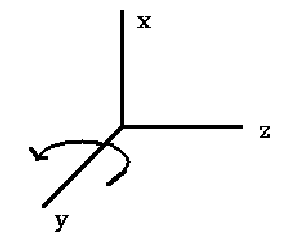Figure 16: 绕y轴旋转

$\begin{cases} z' = z \cos \theta - x \sin \theta \\ x' = z \sin \theta + z \cos \theta \\ y' = y \end{cases}$

$\left( \begin{array}{c} x' \\ y' \\ z' \\ 1 \end{array} \right) = \left( \begin{array}{cccc} \cos \theta & 0 & \sin \theta & 0 \\ 0 & 1 & 0 & 0 \\ -\sin \theta & 0 & \cos \theta & 0 \\ 0 & 0 & 0 & 1 \\ \end{array} \right) \left( \begin{array}{c} x \\ y \\ z \\ 1 \end{array} \right)$

### 4.3 绕任意轴的旋转

$\left( \begin{array}{c} x'_p \\ y'_p \\ z'_p \\ 1 \end{array} \right) = R_{ab}(\theta) \left( \begin{array}{c} x_p \\ y_p \\ z_p \\ 1 \end{array} \right)$

(1) 将A点移到坐标原点。
(2) 使AB分别绕X轴、Y轴旋转适当角度与Z轴重合。
(3) 将AB绕Z轴旋转 $$\theta$$ 角。
(4) 作上述变换的逆操作，使AB回到原来位置。

### 4.4 Yaw, Pitch, Roll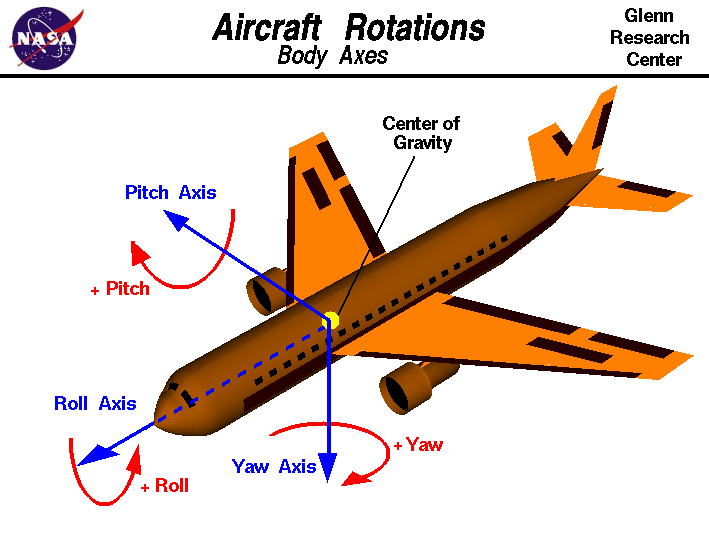Figure 17: Yaw, Pitch, Roll及其“正方向”

Yaw：航向；Pitch：俯仰；Roll：横滚。 如图 18, 19, 20 所示。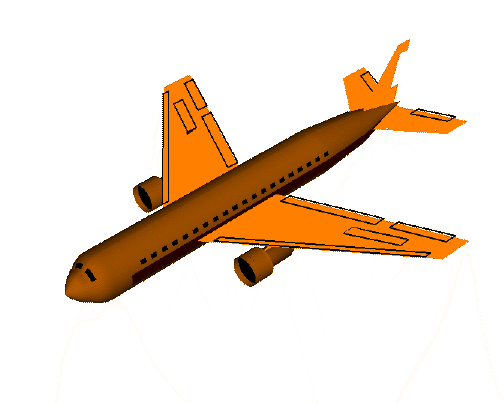Figure 18: Yaw图示（gif动画）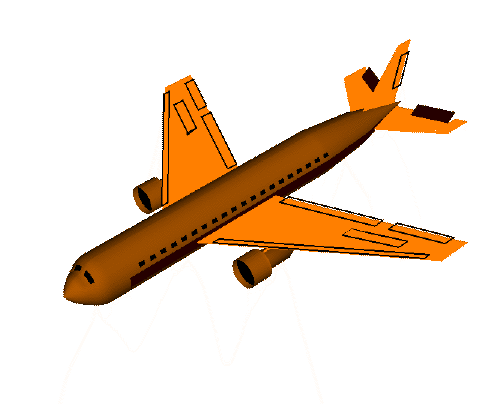Figure 19: Pitch图示（gif动画）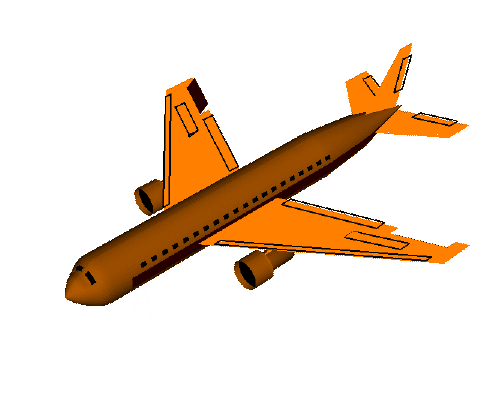Figure 20: Roll图示（gif动画）

### 4.5 用Quaternion表示三维旋转

$\begin{cases} q_0 = \cos(\theta/2) \\ q_1 = \sin(\theta/2) \cos(\phi_x) \\ q_2 = \sin(\theta/2) \cos(\phi_y) \\ q_3 = \sin(\theta/2) \cos(\phi_z) \end{cases}$

Principles of Robot Motion: Theory, Algorithms, and Implementation. Appendix E.4 Quaternions
https://en.wikipedia.org/wiki/Quaternions_and_spatial_rotation

Created: <2016-06-05 Sun 00:00>

Last updated: <2018-01-02 Tue 10:35>

Creator: Emacs 25.3.1 (Org mode 9.1.4)